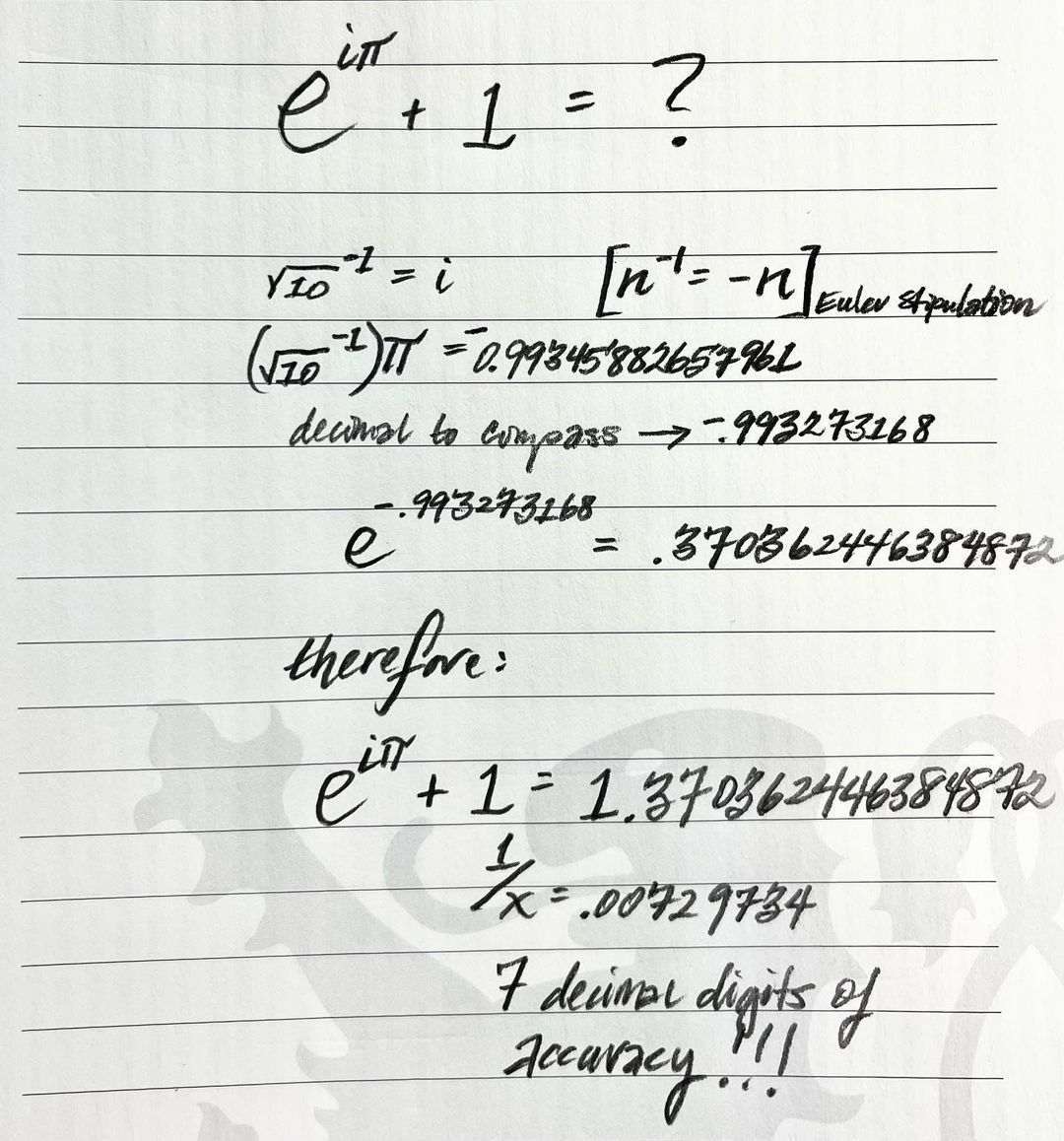### NEW (re) DISCOVERY: e^(iπ) + 1 = LIGHT? The Imaginary Number as the Mathematical Key to the Fifth Dimension.

Root10 is the only value that is able to square its reciprocal. Why? Because Root10 = 3.16227766 and it’s inverse (reciprocal = 1/x) is numerically equivalent = .316227766 infinitely. Since Leonard Euler himself defined n^-1 = -n in his stipulations applicable to the imaginary number ‘i’ this is the only number capable of satisfying this condition and “reciprocal-ly squaring” itself. Its numerical representation is both identical and positive while its reciprocal holds the negative charge polarity required to create the negative number Cartesian Quadrants (currently have 1 ‘Real Number’ plane and 3 Imaginary Number (or ‘Complex’ Planes).Now, what happens if we apply Root10^-1 as the value for ‘i’ in the famous Euler Identity Equation: (e^iπ)+1 = 0? Well actually, it appears that by substituting ‘i’ with the value of (root10^-1) and a conversion to compass values (Base60–SWIPE to see the Decimal to Compass Conversion Calculator screenshot), the answer is no longer ZERO but rather: 1.37036 (1/x = .0072973), the precise value of the Fine Structure Constant (to an astounding 7 decimal digits of accuracy) which defines the very separation of Light from Darkness?!

The Fine Structure Constant a^-1 = 137.036 (1/x = .0072973) and defines the threshold excitation energy required for an electron to emit a photon or absorb (time) it and jump to a higher electron shell.

For a more detailed explanation of this discovery and that the Imaginary Number (Complex Plane) defines the Fifth Dimension, go to my Telegram (link in Profile). And btw, all scales and all numbers ‘work’ across all Cartesian Planes (quadrants) using this value for the Imaginary Number. (The Imaginary Number Root10 x Root10^-1 = -1). The Imaginary Numbers form involuting Tesseracts and the evidence is demonstrated geometrically. I am almost exclusively posting my new geometric/number theory discoveries there these days.

And yes, we will be publishing this as a white paper. Stay tuned. #imaginarynumbersarethefifthdimension